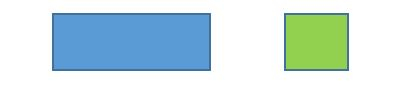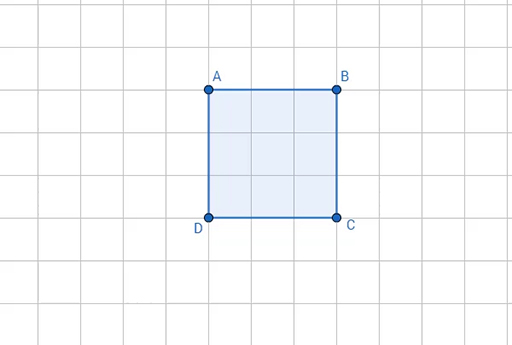Education & Development

### Become an OU studentTeaching mathematics

Start this free course now. Just create an account and sign in. Enrol and complete the course for a free statement of participation or digital badge if available.

# 1.2 Defining shapes using flexible definitions

In this section you will consider how more flexible definitions of shapes can be used to include other kinds of shapes.

Being able to write a definition of a shape is an example of analytical thinking. As learners’ reasoning develops, they are able to define shapes in different ways and to develop definitions which are more inclusive. As an example of this, consider the rectangle and the square (Figure 5).Figure 5 A rectangle and a square

What happens if you were to take one side of the square and pull it? The following video shows this happening.

Download this video clip.Video player: A square being ‘pulled’ into a rectangleA square being ‘pulled’ into a rectangle
Interactive feature not available in single page view (see it in standard view).

You have just watched a square being pulled into a rectangle. Might a square be thought of as a special kind of rectangle? (In mathematics we call this ‘a special case’.)

## Activity 4 Writing definitions

Timing: Allow 5 minutes

Write down a definition of the rectangle.

Write down a definition of the square.

## Activity 5 More definitions

Timing: Allow 10 minutes

Below are two definitions of a rectangle, with a subtle difference between them, and one definition of a square.

Is it possible to include the definition of a square into either of the two definitions of a rectangle?

Definition 1 of a rectangle

A rectangle has four sides and four right angles. It has two longer sides of the same length, which are opposite each other, and two shorter sides of the same length which are opposite each other.

Definition 2 of a rectangle

A rectangle has four sides and four right angles. It has two pairs of equal sides which are opposite each other.

Definition of a square

A square has four sides which are the same length and four right angles.

### Discussion

The definition of a square cannot be included in definition 1 because its definition of a rectangle stipulates that there are two sizes of equal sides: the longer sides and the shorter sides.

The definition of a square can be included in definition 2 because its definition of a rectangle says that there are two pairs of equal and opposite sides. This also includes the case when these two pairs of equal sides are also equal to each other, in which case the rectangle is also a square.

The understanding that some classes of shapes can be included in other classes of shapes is called the concept of inclusion. So, for example, squares can be seen to be special rectangles. However, in order to accept the concept that squares are special rectangles, a more inclusive definition of the rectangle, such as definition 2, needs to be used.

## Activity 6 Including some shapes as special cases of other shapes

Timing: Allow 5 minutes

Try to identify other inclusive relationships. For example, is there a definition of the kite which would include the rhombus? Can rectangles be thought of as special cases of the parallelogram?